# Factors And Multiples Worksheet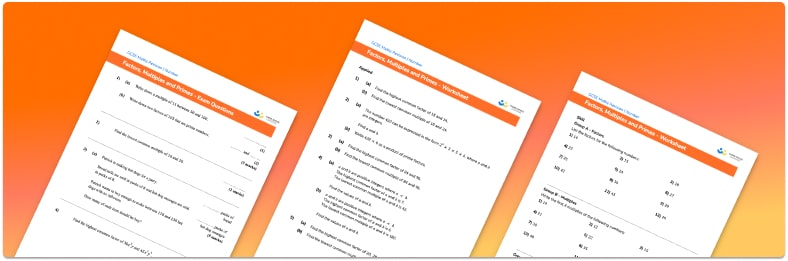• Section 1 of the factors and multiples worksheet contains 36 skills-based factors and multiples questions, in 3 groups to support differentiation
• Section 2 contains 5 applied factors and multiples questions with a mix of worded problems and deeper problem solving questions
• Section 3 contains 4 foundation and higher level GCSE exam style factors and multiples questions
• Answers and a mark scheme for all factors and multiples questions are provided
• Questions follow variation theory with plenty of opportunities for students to work independently at their own level
• All questions created by fully qualified expert secondary maths teachers
• Suitable for GCSE maths revision for AQA, OCR and Edexcel exam boards

• This field is for validation purposes and should be left unchanged.

You can unsubscribe at any time (each email we send will contain an easy way to unsubscribe). To find out more about how we use your data, see our privacy policy.

### Factors and multiples at a glance

Factors are numbers that divide exactly into other numbers. For example, the factors of 12 are 1, 2, 3, 4, 6 and 12. When finding factors of a given number, it can be helpful to think of factor pairs as this will help to ensure we don’t miss any factors. It is important to remember that 1 and the number itself are factors of a number. The highest common factor (HCF/greatest common factor/GCF) of two numbers is the biggest number that is a factor of both.

Multiples are the numbers you get when you multiply a number by any integer. For example, the multiples of 5 are 5, 10, 15, 20, 25 and so on. The lowest common multiple (LCM or least common multiple) of two different numbers is the smallest number that is a multiple of both.

Prime numbers are numbers that only have two factors: themselves and 1. The first few prime numbers are 2, 3, 5, 7 and 11. Numbers that have more than two factors are called composite numbers and any composite number can be written as a product of two or more prime numbers. To find the prime factors of a number we can use a factor tree. The prime factorisation of two or more numbers can be used to find the HCF and LCM of those numbers.

It is helpful if students are confident with multiplication and division facts as well as working with square numbers, cube numbers and higher powers when covering these topics.

Looking forward, students can then progress to additional number worksheets, for example aorFor more teaching and learning support on Number our GCSE maths lessons provide step by step support for all GCSE maths concepts.

## Related worksheets

HCF and LCM Worksheet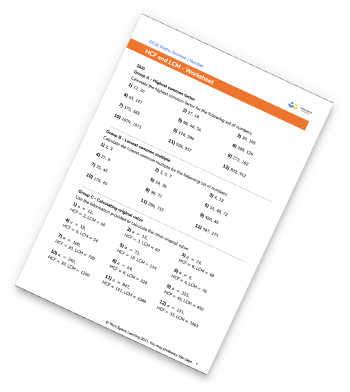Factor Trees Worksheets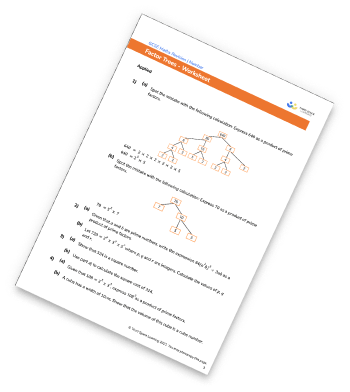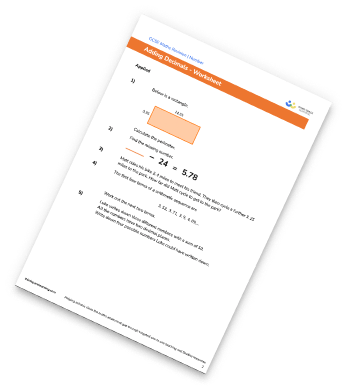Subtracting decimals worksheet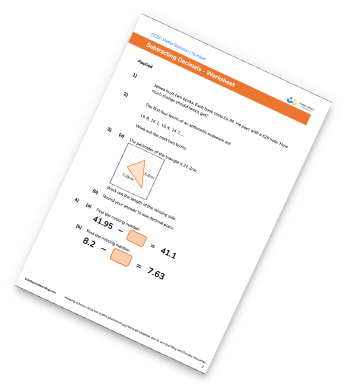## Do you have KS4 students who need more focused attention to succeed at GCSE?There will be students in your class who require individual attention to help them succeed in their maths GCSEs. In a class of 30, it’s not always easy to provide.

Help your students feel confident with exam-style questions and the strategies they’ll need to answer them correctly with our dedicated GCSE maths revision programme.

Lessons are selected to provide support where each student needs it most, and specially-trained GCSE maths tutors adapt the pitch and pace of each lesson. This ensures a personalised revision programme that raises grades and boosts confidence.

Find out more